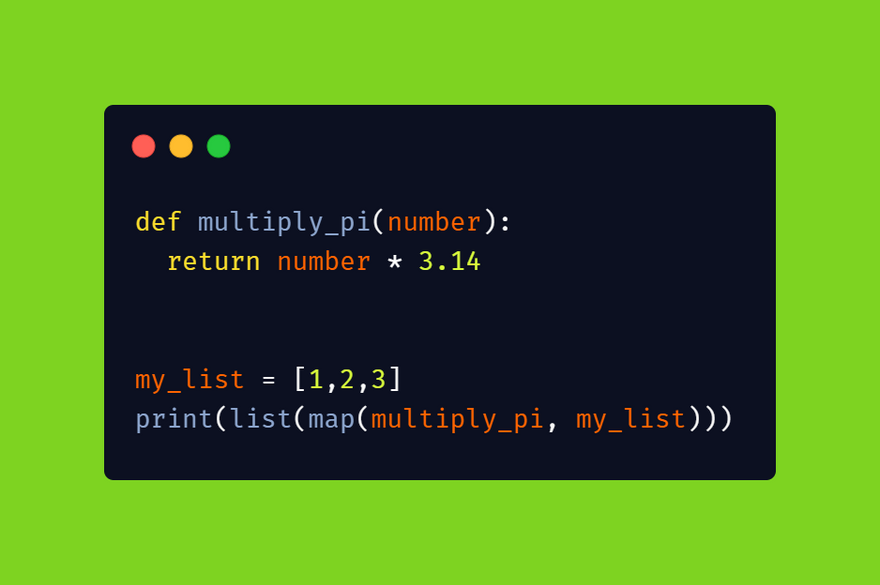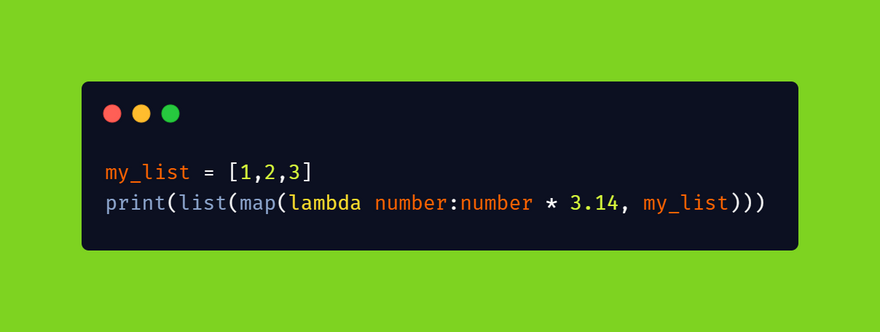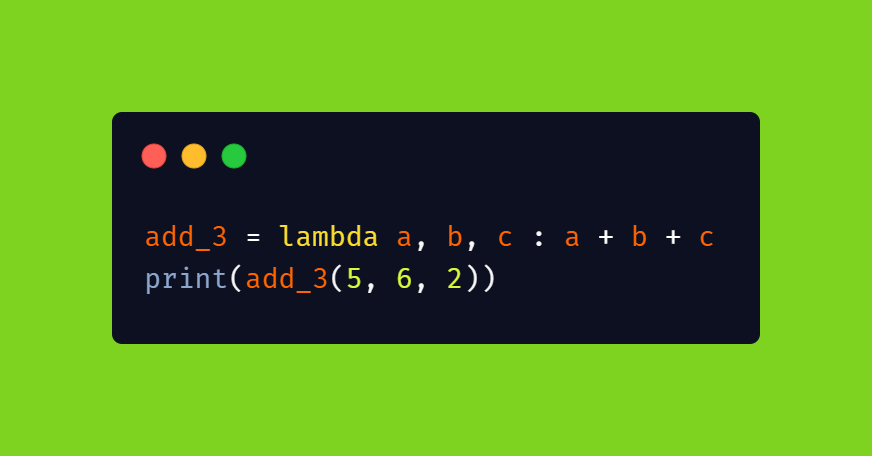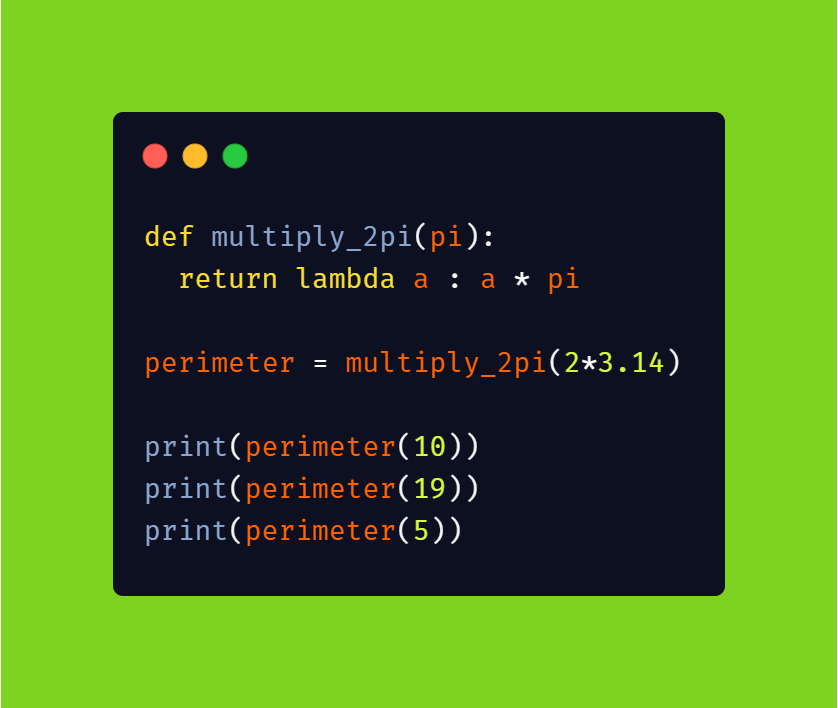## CodeNewbie Community 🌱

Aswin Barath

Posted on • Originally published at dev.to

# Lambda expressions in python

Lambda expressions in python are one-time anonymous functions which we don't need more than once.

Consider the following example where we use a function to multiply a list of numbers with the mathematical constant pi:Output:

``````[3.14, 6.28, 9.42]
``````

What if I say that we could do all of this in one line.
Well, it turns out we can do it.
We can convert the above example into a single line of code using Lambda expressions.Output:

``````[3.14, 6.28, 9.42]
``````

## Lambda function

Syntax: `lambda arguments : expression`

• A lambda function can take any number of arguments, but can only have one expression:Output:

``````94
``````

## Why do we need to use Lambda functions?

• Well, it contributes to the code by reducing the number of lines of functions which we may use only once.
• To take it to the next level, we can return an anonymous function inside another example:Output:

``````62.800000000000004
119.32000000000001
31.400000000000002
``````

As you can see, we got to use the same function to calculate different values.
You get to create a function definition that takes one argument, and that argument will be multiplied with an unknown number (which you can specify in the future).

That's the power of lambda expressions.